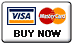Sacred Geometry aka Gematria

The English alphabet has 26 letters and the number system for these letters are 1,2,3,4,5,6,7,6,5,4,3,2,1 for the first 13 letters A-M and the number system for the last 13 letters are 1,2,3,4,5,6,7,6,5,4,3,2,1, N-Z. Notice that the number 7 is in the middle of the each sequence. Origin of this cypher may have come from Freemasons, who could have adopted it from the Kabbala or Chaldean numerology systems. ( updated origin info )

Gematria is a method of interpreting words,based on numerical values of letters.(Greek geometria) Every thing that is created by man involves mathematics, geometry aka Sacred Geometry (Pi). I find it interesting the words Gematria and Geometria when added using the cipher equal 70 and if we use decimal parity 7+0 = 7. Why is this significant, well if you spell out 7 seven it equals 22 and 22\7 = 3.142.. which is the closest known fraction to Pi (P3,I5 ) = 8. This writer uses simple math aka addition, subtraction, multiplication and division in his work.Connecting To Sophia-Gaia By Way Of Gematria The Story Of Creation / The Journey Of My Soul